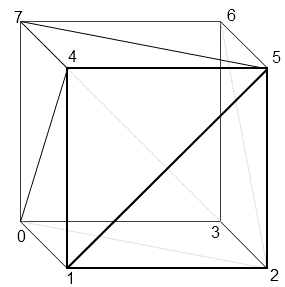## 2014年2月25日 星期二

### WebGL Demo 01: 3D transformation and Lambert lighting

This demo would talk about some 3D computer graphics background knowledge, and to show how to use WebGL to do 3D transformation and Lambert lighting.

Using maximus.js, author by Ellis Mu

In this demo, I develop a WebGL framework named maximus.js to proof the functions which been implemented by three.js. I choose the same 3d coordinate system as three.js, which is Y-up and right-hand system.

1. Vertex buffer
In the 3d graphics pipeline, the data format of primitive which GPU can accept, They are vertex buffer and index buffer.

What is vertex buffer? Each primitive must be constructed by vertices. In the case of cube, we create a mesh which has 24 vertices, because it has six faces and per face has four vertices.
``` _cubeVertexBuffer = gl.createBuffer();  // create a buffer
gl.bindBuffer( gl.ARRAY_BUFFER, _cubeVertexBuffer ); // bind this buffer as vertex buffer

var vertices = [             // Create these vertices
// Front face
-1.0, -1.0, 1.0, 1.0, 0.0, 0.0, 1.0, 0.0, 0.0, 1.0,
1.0, -1.0, 1.0,  0.0, 1.0, 0.0, 1.0, 0.0, 0.0, 1.0,
1.0, 1.0, 1.0, 0.0, 0.0, 1.0, 1.0, 0.0, 0.0, 1.0,
-1.0, 1.0, 1.0, 1.0, 1.0, 0.0, 1.0, 0.0, 0.0, 1.0,....
];

gl.bufferData( gl.ARRAY_BUFFER, new Float32Array(vertices), gl.STATIC_DRAW ); // And then uploading these vertices data to vertex buffer```
2. Index buffer
Index buffer can help us reduce the usage number of vertices. For example, when we want to draw a quad which has two triangle, so we need to define six vertices. But while using index buffer, we just need to record 4 vertices of this quad, and use indices to define as two primitives. This approach can help us reduce massive data while using high-polygon models.In the index buffer, we record [0,1,2,2,3,0] to define two primitives that will search the no.0,1,2,3 order in the vertex data in the GPU primitive assembly stage.

3. 3D transformation
In the 3D space, we must use lots of Matrix transformation. In this figure, we describe we want to transform our objects to the near clipping plane which is the final 2D screen space. Every model has its own modelToWorld matrix to transform to world space. While a camera moving in a 3D scene, the camera has its own camera view matrix, and the screen space define the projection matrix. Therefore, we use this formula to transform model from object space to screen space.

Modelscreen = obj2worldMtx X world2cameraMtx X projectionMtx X Modelobject

Graphics pipeline has several generation, From fixed-function, shader model pipeline, deferred rendering, to physical-based lighting. In this WebGL demo, this is a basic shader model pipeline. We have to send vertex and fragment shader to link program. There are three types of variable we need to know.
• Attribute
Attribute means the vertex input from vertex buffer data. This has its own order based on vertexPositionAttribute.
• Varying
Varying means the vertex output of vertex shader, that will be interpolated by Rasterizer and be sent to pixel shader.
• Uniform
Uniform variable are be set from your application program by uniformMatrix4fv or uniform4fv
5. Lighting
Lambert lighting is a basic lighting algorithm in the 3D computer graphics. It just has to compute the dot of light vector and pixel normal vector. If the angle >= 90, that means no lighting, else it has lighting.

6. Using three.js
Finally, I write the same demo by using three.js. It becomes so simple. We just need to the several lines code like this.
```              function init() {
renderer = new THREE.WebGLRenderer();
renderer.setSize( 400, 300 );
renderer.setClearColor( 0x000000, 1.0 );  // set frame buffer clear color
document.body.appendChild( renderer.domElement );

scene = new THREE.Scene();
camera = new THREE.PerspectiveCamera( 45, 400/300, 0.1, 10000 ); // Projection matrix setup

camera.position.set( 0, 0, 20 );  // Setup camera
camera.lookAt( scene.position );

var geometry = new THREE.CubeGeometry( 5, 5, 5 );  // Create cube geometry and material
var material = new THREE.MeshLambertMaterial( { color: 0xFF0000 } );
mesh = new THREE.Mesh( geometry, material );

scene.add( mesh );  // Add cube to scene manager

var light = new THREE.DirectionalLight( 0xFFFFFF, 1.0 ); // Create lighting
light.position.set( 10, 0, 10 );
}

function updateFrame() {
requestAnimationFrame( updateFrame );
mesh.rotation.y += THREE.Math.degToRad( 5 ); // Rotate the cube

render();
}

function render() {
renderer.render( scene, camera );  // render the meshes of the scene using this camera viewport.
}
```

Using three.js

Github: https://github.com/DaoshengMu/Maximus-WebGL